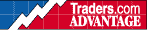HOT TOPICS LIST

INDICATORS LIST

LIST OF TOPICS

# Ease of Movement

04/02/00 02:23:42 PM
by Jason K. Hutson

Ease of Movement

Security:   N/A
Position:   N/A

 First, calculate the midpoint movement (MPM):MPM TH TL YH YL = + - +2 2whereTH = Today's highYH = Yesterday's highTL = Today's lowYL = Yesterday's low If, for example, yesterday the stock had a high of 32 and a low of 31, its midpoint would be 31 -1/2. Iftoday it had a high of 34 and a low of 32, then its midpoint would be 33. The midpoint move would be 33minus 31 1/2, or 1 1/2. The next step is to calculate the box ratio. The box is the Equivolume box, which uses the day 'shigh-to-low price range for the vertical axis and the volume for the horizontal axis. Use a number ofincrements to represent volume %97 for example, 10,000 shares equal 1 unit. A 55,000-share day wouldhave 5.5 units. For the height of the box, use 1/8 of a point for 1 unit. If a stock had a high of 24 and alow of 23- 1/8, the number of units would be 7 units. Divide the volume units by the range units for thebox ratio. EMV=(Midpo int move)/(Box ratio)

Jason K. Hutson

Traders.com Staff Writer. Enjoys trendlines, support and resistance, moving averages, RSI, MACD, ADX, Bollinger bands, parabolic SAR, chart formations, and volume analysis.

 Comments or Questions? Article Usefulness 5 (most useful) 4 3 2 1 (least useful)